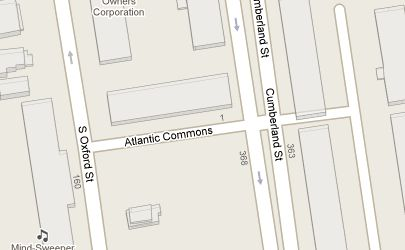# 12. Spatial Relationships Exercises¶

Here’s a reminder of the functions we saw in the last section. They should be useful for the exercises!

• sum(expression) aggregate to return a sum for a set of records

• count(expression) aggregate to return the size of a set of records

• ST_Contains(geometry A, geometry B) returns true if geometry A contains geometry B

• ST_Crosses(geometry A, geometry B) returns true if geometry A crosses geometry B

• ST_Disjoint(geometry A , geometry B) returns true if the geometries do not “spatially intersect”

• ST_Distance(geometry A, geometry B) returns the minimum distance between geometry A and geometry B

• ST_DWithin(geometry A, geometry B, radius) returns true if geometry A is radius distance or less from geometry B

• ST_Equals(geometry A, geometry B) returns true if geometry A is the same as geometry B

• ST_Intersects(geometry A, geometry B) returns true if geometry A intersects geometry B

• ST_Overlaps(geometry A, geometry B) returns true if geometry A and geometry B share space, but are not completely contained by each other.

• ST_Touches(geometry A, geometry B) returns true if the boundary of geometry A touches geometry B

• ST_Within(geometry A, geometry B) returns true if geometry A is within geometry B

Also remember the tables we have available:

• `nyc_census_blocks`

• blkid, popn_total, boroname, geom

• `nyc_streets`

• name, type, geom

• `nyc_subway_stations`

• name, geom

• `nyc_neighborhoods`

• name, boroname, geom

## 12.1. Exercises¶

• “What is the geometry value for the street named ‘Atlantic Commons’?”

```SELECT ST_AsText(geom)
FROM nyc_streets
WHERE name = 'Atlantic Commons';
```
```MULTILINESTRING((586781.701577724 4504202.15314339,586863.51964484 4504215.9881701))
```
• “What neighborhood and borough is Atlantic Commons in?”

```SELECT name, boroname
FROM nyc_neighborhoods
WHERE ST_Intersects(
geom,
ST_GeomFromText('LINESTRING(586782 4504202,586864 4504216)', 26918)
);
```
```    name    | boroname
------------+----------
Fort Green | Brooklyn
```

Note

“Hey, why did you change from a ‘MULTILINESTRING’ to a ‘LINESTRING’?” Spatially they describe the same shape, so going from a single-item multi-geometry to a singleton saves a few keystrokes.

More importantly, we also rounded the coordinates to make them easier to read, which does actually change results: we couldn’t use the ST_Touches() predicate to find out which roads join Atlantic Commons, because the coordinates are not exactly the same anymore.

• “What streets does Atlantic Commons join with?”

```SELECT name
FROM nyc_streets
WHERE ST_DWithin(
geom,
ST_GeomFromText('LINESTRING(586782 4504202,586864 4504216)', 26918),
0.1
);
```
```     name
------------------
Cumberland St
Atlantic Commons
```• “Approximately how many people live on (within 50 meters of) Atlantic Commons?”

```SELECT Sum(popn_total)
FROM nyc_census_blocks
WHERE ST_DWithin(
geom,
ST_GeomFromText('LINESTRING(586782 4504202,586864 4504216)', 26918),
50
);
```
```1438
```